# 2.1. Resistor circuits¶

## 2.1.1. Objective¶

The objective of this activity is to brush up on your existing knowledge about Kirchhoff’s laws and expand on that knowledge by showing how they can be applied in resistor circuits. A secondary outcome will be a preliminary understanding of the Red Pitaya STEMlab hardware and software - test & measurements applications.

## 2.1.2. Background¶

We will kick this lesson off by taking a look at the basic equation, you will need to know if you ever wanted to tinker with electronics.

$U=R \cdot I$

This equation is the backbone of resistor circuits. Note that “a resistor circuit” is every circuit that you leave untouched for long enough, if there are no active elements. The second equation that comes in handy in such circuits is the one, which describes power dissipation on any resistor.

$P=U \cdot I$

Even though you can always determine voltage drop across, and current flowing through any resistor, it often comes handy to remember two more equations, in which voltage drop or current is substituted for its function of the other two values.

$P=I^2 \cdot R = U^2/R$

With this out of the way, we can move on to…

## 2.1.3. Kirchhoff’s laws¶

To solve a circuit that consists of more than one component, you will need to know two more things, also known as Kirchhoff’s laws. Kirchhoff’s current law (KCL):

“The algebraic sum of currents in a network of conductors meeting at a point is zero. “

To put it simply, any current entering a node must also leave it. If the first law talks about current, logic would suggest that he second will be about voltage (KVL).

“The directed sum of the potential differences (voltages) around any closed loop is zero.”

If you have no idea what Mr. Kirchhoff meant, when he came up with his laws, don’t worry. It will become crystal clear when we take a look at examples later on. But first, we will take a look at a few equations and facts, that tend to make our lives easier when solving resistor circuits.

## 2.1.4. Some equations and facts¶

When you only have one voltage source, you can always calculate any voltage drop or current by simplifying the circuit using the following two equations for equivalent substitute resistor.

$R_{S_{series}} = R_1 + R_2$
$\frac{R_{S_{parallel}}}{1} = \frac{1}{R_1} + \frac{1}{R_2}$

Once you reach the circuit that only consists of one voltage (or current) source and one substitute resistor, you can trace the simplification steps backwards, calculating branch voltages and currents as you go along. Here you will need to know what happens to currents and voltages when you branch out. If substitute resistor is split into multiple parallel resistors, voltage drop across them remains the same. In fact, voltage drop between two nodes must remain the same no matter how you get between them (this comes from KVL). Current often behaves somewhat inversely to voltage, and as such, it remains the unchanged when a substitute resistor is split into multiple series resistors. If substitute resistor is split into series resistors, voltage is split among them proportionally to their resistance. We won’t go into details why that is, so an equation will have to suffice.

$U_{R_x}=U_{R_{S_{series}}} \cdot \frac{R_x}{R_{S_{series}}}$

It will come as no surprise that current is split proportionally to resistor’s resistance when it’s split into multiple parallel ones. Here’s the equations.

$I_{R_x} = I_{R_{S_{parallel}}} \cdot \frac{R_{S_{series}} - R_x}{R_{S_{series}}}$

It will come as no surprise, that the lesser the resistance, the more current wants to flow through it, and the greater the resistance, the bigger the voltage drop. Before we move on to measurements, two more things to remember. When solving circuits in steady state, capacitors act as an open circuit, and inductors act as a short circuit. Consider this as a useful side note and move on.

## 2.1.5. Practical example¶

You might be disappointed to learn that the circuit used in this example will be different from the one that was shown in the video. This is so that you can easily solve it by either using Kirchhoff’s laws or by substitution.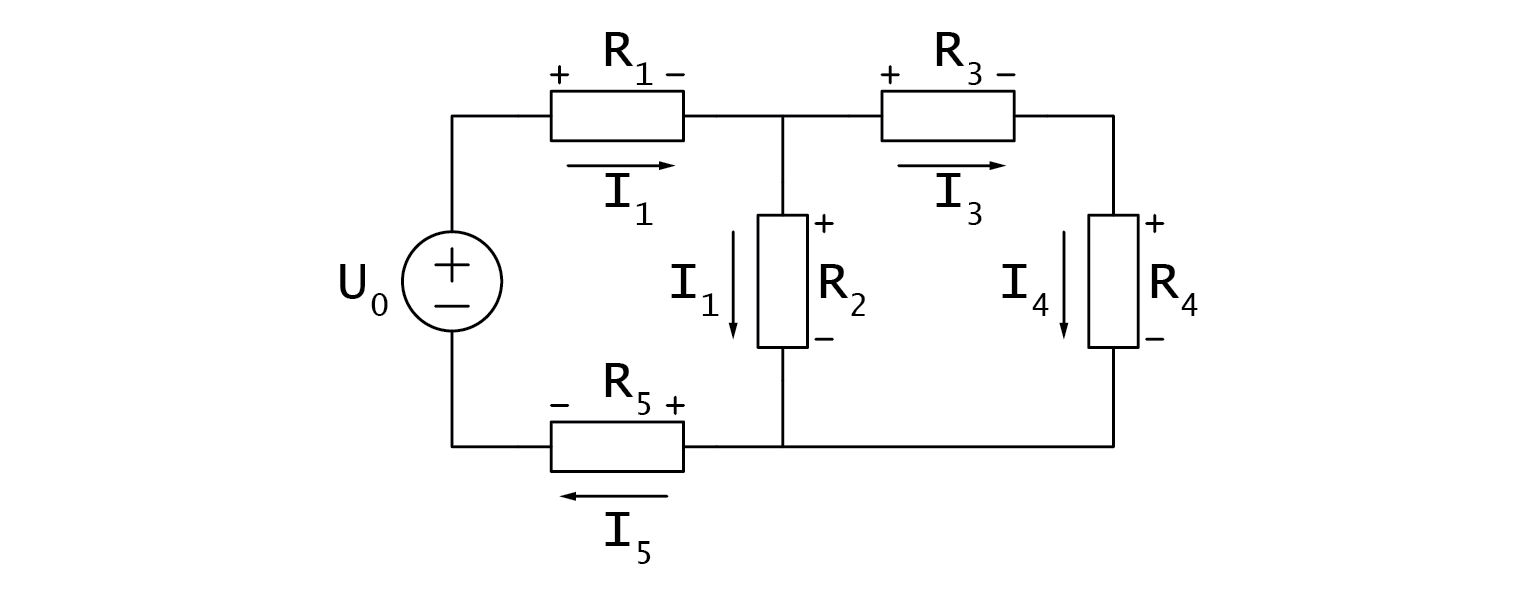Let’s assume that we are tasked by calculating voltage drop, current, and power dissipation on $$R_2$$. We’ll first tackle this problem by substitution. Note that I am using “|” symbol to represent equivalent resistance of parallel resistor.To put it into numbers:

$I_0=\frac{U_0}{R_{S_{total}}} = \frac{U_0}{(R_1+(R_2 |(R_3+R_4))+R_5 )}=...$
$U_{R_2} = U_0 \cdot \frac{R_2 |(R_3+R_4)}{R_{S_{total}}} =...$
$I_{R_2} = \frac{U_{R_2}}{R_2} =...$
$P_{R_2} = U_{R_2} \cdot I_{R_2}=...$

Note that there was no need to calculate all voltage drops and currents to reach our goal. Next we will take a look at the more academic method. First we have to analyse the circuit. It has two branching nodes, which means we will need two node equations (KCL). We can also find three distinct current loops, and we will need one loop equation less (KVL).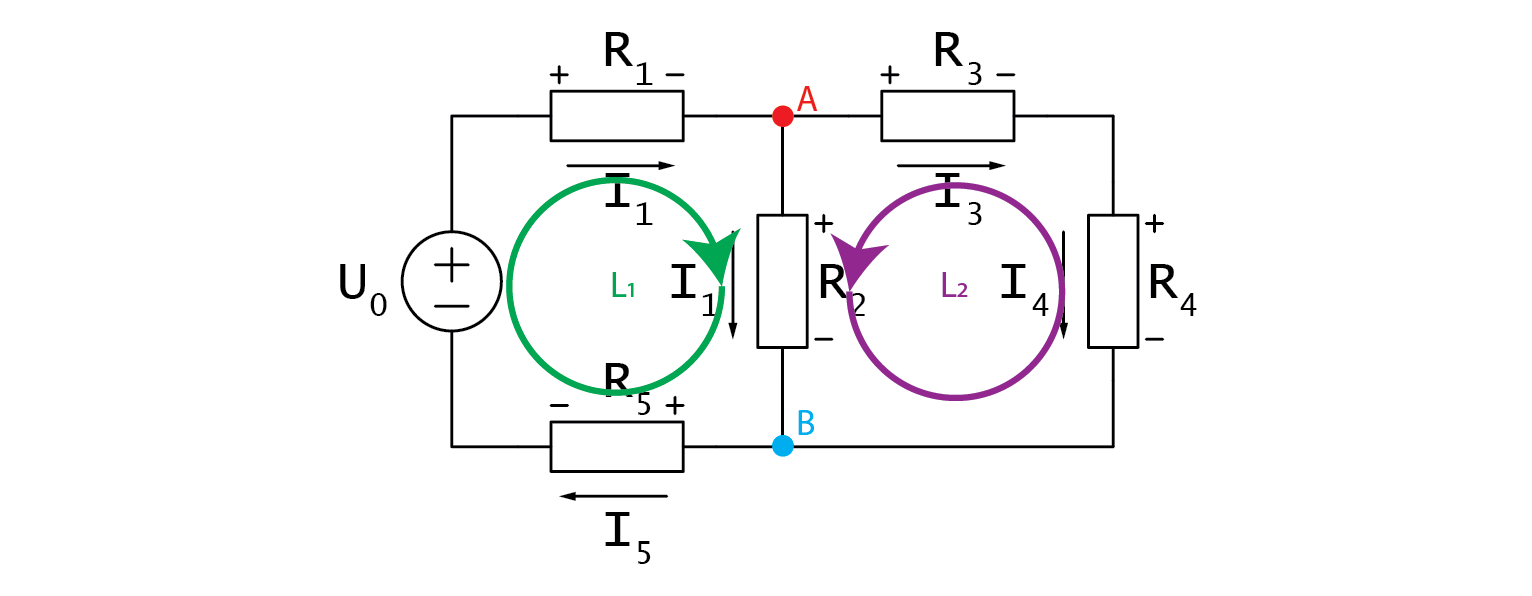Let’s write them down.

$A: \;\;\; I_2+I_3-I_1=0$
$B: \;\;\; I_5-I_2-I_4=0$

I would like to mention that you should immediately see from the schematic that we have redundantly many currents. $$I_s$$, $$I_1$$, and $$I_5$$ are exactly the same, so are $$I_3$$ and $$I_4$$. Moving along the KVL loops, we must be adding any voltage that we hit from the + side, and subtracting those that we hit from the -.

$L1: \;\;\; U_{R_1} + U_{R_2} + U_{R_5} - U_0 = 0$
$L2: \;\;\; U_{R_3} + U_{R_4} - U_{R_2} = 0$

Let’s first take a look at what we can do with the two node equations. First we can substitute redundant currents in B with the ones from A:

$I_5 - I_2 - I_4 = 0 \rightarrow I_2 + I_3 - I_1 = 0$

Keen eyed among you will notice that after this transformation, equations A and B are the same equation. That makes things easy as we can simply express one of the currents as a function of the other two and move on to solving voltage equations.

$I_1 = I_2 + I_3$
$equation\;A$

Voltage drops in voltage loops should be written as products of currents and respective resistances.

$U_{R_3} + U_{R_4} - U_{R_2} = 0$
$I_3R_3 + I_3R_4 = I_2R_2$
$I_3(R_3 + R_4) = I_2R_2$
$I_2 = I_3\frac{R_3+R_4}{R_2}$
$equation\;B$

This one wasn’t too bad, let’s take a look at the other voltage loop:

$U_{R_1} + U_{R_2}+U_{R_5}-U_0=0$
$U_{R_1}+U_{R_2}+U_{R_5}-U_0=0$

Unlike before, we are dealing with three distinct currents. This can be solved by plugging in $$equation\;A$$, and we get:

$(I_2+I_3)R_1+I_2 R_2+(I_2+I_3)R_5=U_0$
$I_2 (R_1+R_2+R_5 )+I_3 (R_1+R_5 )=U_0$
$(I_3 \frac{R_3+R_4}{R_2})(R_1+R_2+R_5 )+I_3 (R_1+R_5 )=U_0$
$I_3=\frac{U_0}{\frac{R_3+R_4}{R_2}(R_1+R_2+R_5 )+(R_1+R_5 ) }$

And there you go, we now have an equation for $$I_3$$ that only relies on known constants. We only need to plug the values in and from there on, dominos will fall. Plugging $$I_3$$ into $$equation\;B$$ yields $$I_2$$. From there on, $$equation\;A$$ gives us $$I_1$$ and all of a sudden all currents are known. Lastly we can use $$equation\;L1$$ to get any voltage drop we desire and all left to do is to calculate the power, which is now one simple multiplication away. Was this more difficult than doing substitutions? Depends on who you ask. I solved the circuit both ways and don’t get me started on how much I hate calculating substitute resistance for parallel resistors. Besides, the second method yields all voltages and currents at once, which is what you will usually tasked with on the exams.

## 2.1.6. Hands on¶

You must have noticed that I shied away from using any numbers in my calculations. Let me tell you that I’ve been at university for such a long time that I’ve forgotten what equations with actual numbers even look like. Jokes aside, I shied away from numbers because, as you saw, we didn’t need them. Also in this hands on part, you will select your own resistors and measure voltages across them. You can select any resistors (but avoid going below 100 ohms so that nothing accidentally gets fried), and build the circuit on a breadboard as shown on the picture below. For U_0 you can choose between Red Pitaya’s supply pins. It can be 3.3 V, 5 V or even -4 V. Anything you fancy!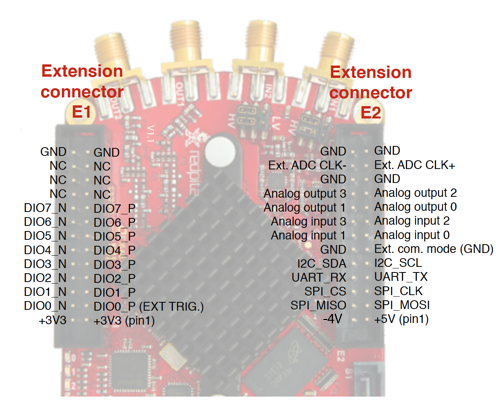With that done, you should hook the probes in 10x mode to Red Pitaya and fire up the oscilloscope app. Don’t forget to set the x10 attenuation in software as well! Since we are dealing with DC signals, you don’t need to hook up the alligator clips (they’re internally connected to Red Pitaya’s GND). You can now measure voltage on any node by connecting a probe to it.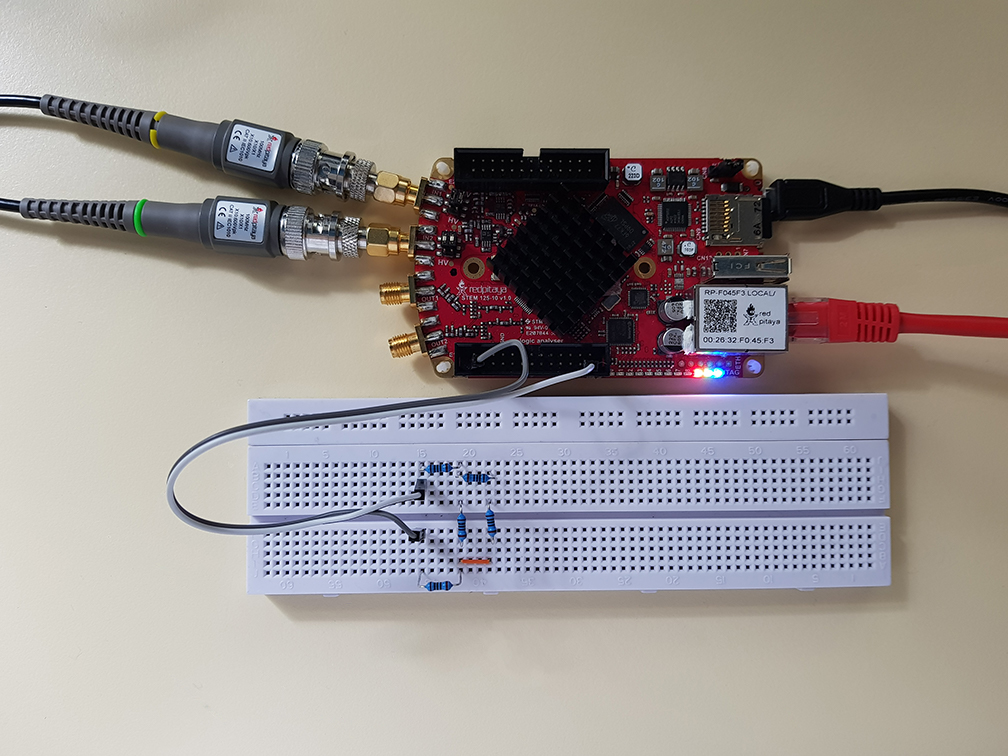One thing you might want to do though, is to set up automatic mean measurements on both channels to make reading voltage easier (MEAS -> Operator = MEAN -> DONE).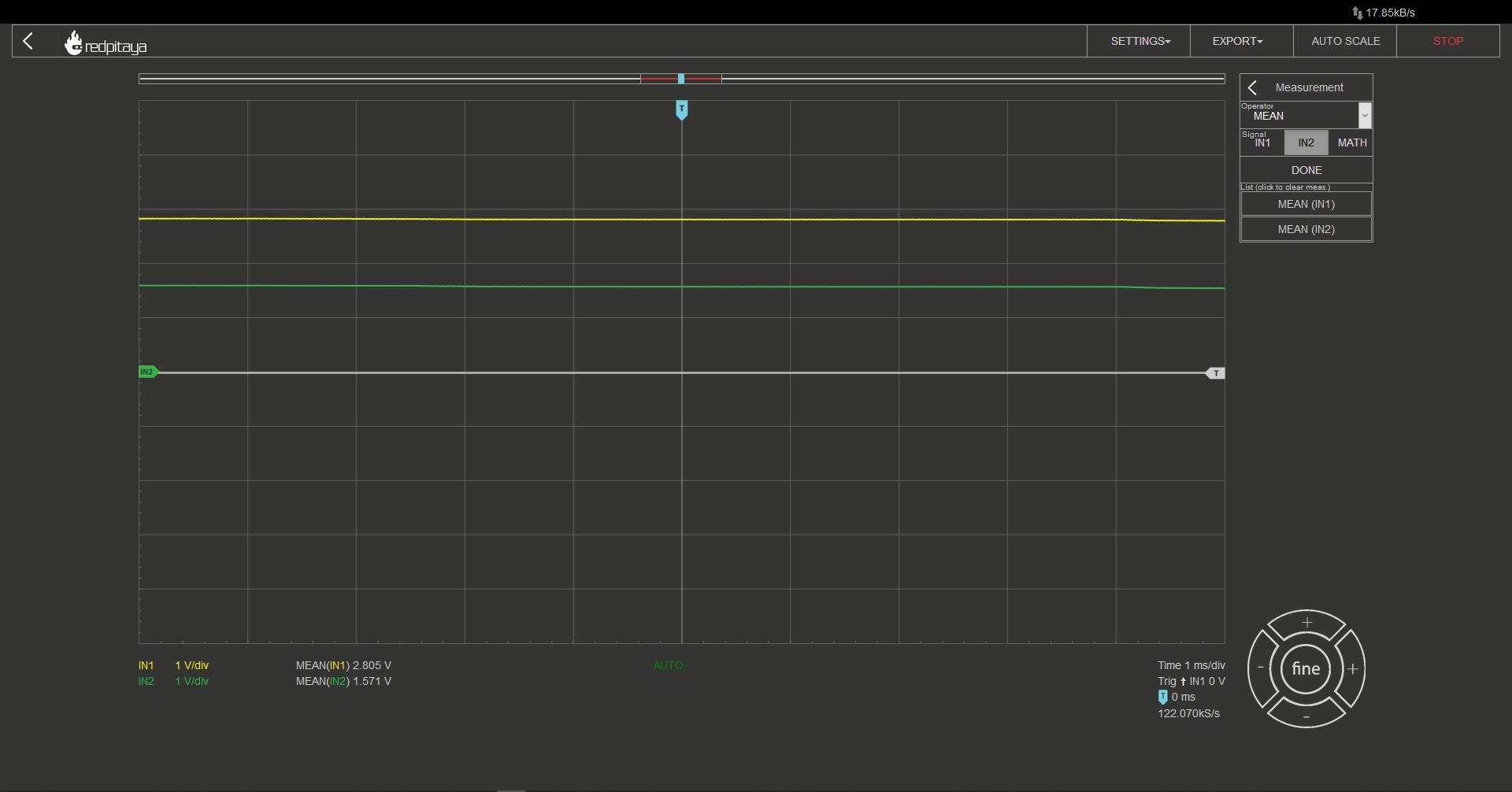I encourage you to build a different circuit. Don’t exceed three branching nodes to keep the calculations simple. Try to calculate voltage drops and compare them with measured values.

Written by Luka Pogačnik

This teaching material was created by Red Pitaya & Zavod 404 in the scope of the Smart4All innovation project.